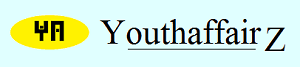March 2015

# Solution

First, let's get all the facts in the proper perspective. The conical container is filled to the brim initially and, so, the soft drink inside takes the shape of the container (that is the cone) and its height is same as the height of the cone. Let the height of the cone be "h" cm, and let its radius be "r" cm. Therefore, h = 12. The value of "r" is not known.

The volume of a right conical cone is (1/3) * (pi * r2 * h).

Therefore, initially, the quantity or volume of soft drink that we have is V1 = (1/3) * (pi * r2 * 12).

Thus, initially, we have V1 = 4 * pi * r2.

Now, 4 cm of the soft drink has been poured into the glass. The soft drink height in the conical container now stands at 8 cm. In a right circular cone, the radius is proportional to the height. Therefore, h/r = H/R where "H" is the new height and "R" is the new radius. But, H = 8. Thus, 12/r = 8/R. So, R = 8r/12 = 2r/3.

So, the volume of soft drink in the conical vessel now is: (1/3) * pi * (2r/3)2 * 8.

So, volume of soft drink in conical container is V2 = (32 * pi * r2)/27.

Quantity of soft drink in the glass is, of course, the difference between the initial quantity and the amount in the conical container, ie V1 - V2.

Let the volume of soft drink in the glass be V3. Then, V3 = V1 - V2.

So, V3 = (4 * pi * r2) - (32 * pi * r2)/27.

So, V3 = (76 * pi * r2)/27.

Let's represent (pi * r2) by the letter "L" for the ease of reading. Therefore, V1 = 4L, V2 = 32L/27, and V3 = 76L/27.

Now, 76L/27 is certainly much greater than 32L/27; it is, in fact, more than double. So, Shomu has received more than double the quantity of soft drink than me! Just let me get my hands on Shomu!

Did I get my calculations right, or am I simply gunning for Shomu for no reason?

As an afterthought, I was wondering if after emptying the soft drink in the conical container the soft drink from Shomu's glass was poured into it, what height would it occupy.

Let's work this out. The volume of soft drink in Shomu's glass is V3 = (76 * pi * r2)/27.

After pouring this into the conical container, the soft drink will take the shape of a cone with, say, height = "H1" and radius = "R1". Its volume will be (1/3) * (pi * R12 * H1).

Therefore, (1/3) * (pi * R12 * H1) = (76 * pi * r2)/27.

So, R12 * H1 = (228 * r2)/27.

Now, as already stated, the radius is proportional to the height in a right circular cone. Therefore, R1/H1 = r/h.

Therefore, R1/H1 = r/12. So, R1 = (r * H1)/12.

Let's substitute and see what we get.

We get [(r * H1)/12]2 * H1 = (228 * r2)/27.

Therefore, (r2 * H13)/144 = (228 * r2)/27.

So, H13 = (228 * 144)/27.

Thus, H13 = 1216.

Therefore, H1 = cube root of 1216 = 10.673, much larger than the 8 cms of soft drink that I got as my share! Shomu has indeed made a fool of me!

• Union Public Service Commission - www.upsc.gov.in
• IIT-Kharagpur - www.iitkgp.ac.in
• Indian Statistical Institute - www.isical.ac.in
• Indian Institute of Technology Madras - www.iitm.ac.in
• Indian Institute of Management, Ahmedabad - www.iimahd.ernet.in
• Indian Institute of Mass Commission - www.iimc.nic.in
• IIT Bombay - www.iitb.ac.in